Courses

# Circular Motion MSQ

## 10 Questions MCQ Test Topic wise Tests for IIT JAM Physics | Circular Motion MSQ

Description
This mock test of Circular Motion MSQ for Physics helps you for every Physics entrance exam. This contains 10 Multiple Choice Questions for Physics Circular Motion MSQ (mcq) to study with solutions a complete question bank. The solved questions answers in this Circular Motion MSQ quiz give you a good mix of easy questions and tough questions. Physics students definitely take this Circular Motion MSQ exercise for a better result in the exam. You can find other Circular Motion MSQ extra questions, long questions & short questions for Physics on EduRev as well by searching above.
*Multiple options can be correct
QUESTION: 1

### One end of a light string of length L is connected to a ball and the other end is connected to a fixed point O. The ball is released from rest at t = 0 with string horizontal and just taut. The ball then moves in vertical circular path as shown. The time taken by ball to go from position A to B is t1 and from B to lowest position C is t2. Let the velocity of ball at B is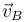and at C is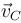respectively. If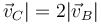then, which of the following is true.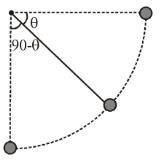Solution: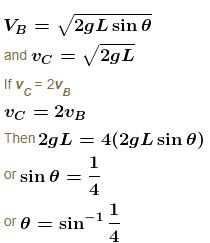Tangential acceleration is at = g cos θ which decrease with time.

Hence the plot of at versus time may be as shown in graph.

Area under graph in time interval t1 = vB – 0 = vB

Area under graph in the time interval t2 = vC – vB = vB

Hence the under graph in time t1 and t2 is same.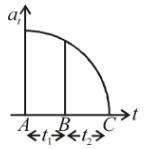∴  t1 < t2

∴ t1 < t2

The correct answers are: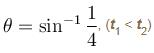*Multiple options can be correct
QUESTION: 2

### A machine, in an amusement park, consists of a cage at the end of one arm, hinged at O. The cage revolves along a vertical circle of radius r (ABCDEFGH) about its hinge O, at constant linear speed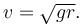The cage is so attached that the man of weight ‘w’ standing on a weighing machine, inside the cage, is always vertical. Then which of the following is/are correct.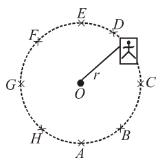Solution:

Let N be the normal reaction (Reading of the weighing machine)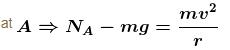Put v ∴  NA – mg = mg
⇒  NA = 2mg = 2W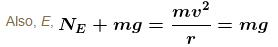hence  NA > NE by 2W
Now at GNG = mg = W = Nc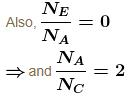The correct answers are: the weight reading at A is greater than the weight reading at E by 2 w, the weight reading at G = w, the ratio of the weight reading at E to that at A = 0, the ratio of the weight reading at A to that at C = 2

QUESTION: 3

### A particle is moving in a circular path. The acceleration and momentum vectors at an instant of time are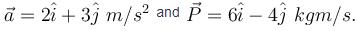Then which of the following statement is incorrect

Solution: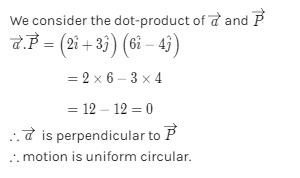*Multiple options can be correct
QUESTION: 4

A car driver going at some speed suddenly finds a wide wall at a distance r. To avoid hitting the wall he should.

Solution:

When he applies brakes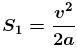if µ is the friction coefficient then a = µg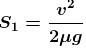when he takes turn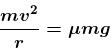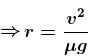Then we can see r < s1 hence driver can hit the wall when he take turn due to insufficient radius of curvature.

The correct answer is: apply the brakes

*Multiple options can be correct
QUESTION: 5

Two particles A and B separated by a distance 2R are moving counter clockwise along the same circular path of radius R each with uniform speed v. At time t = 0. A is given a tangential acceleration of magnitude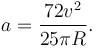Solution:

As when they collide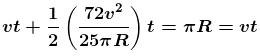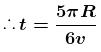Now angle covered by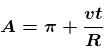Put t ∴ angle covered by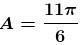The correct answers are: the time lapse for the two bodies to collide is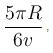the angle covered of A is 11π/6

*Multiple options can be correct
QUESTION: 6

A smooth rod PQ rotates in a horizontal plane about its mid point M which is h = 0.1 m vertically below a fixed point A at a constant angular velocity 14 rad/s. A light elastic string of natural length 0.1 m force constant 1.47 N/cm has one end fixed at A and its other end attached to a ring of mass m = 0.3 kg which is free to slide along the rod. When the ring is stationary relative to rod, then which of following is correct.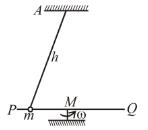Solution: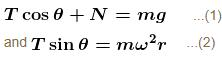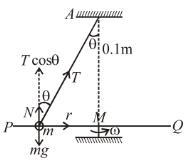but R = Kx
(K = 1.47 × 102 N/m)
Also  r = 0.1 tan θ
put Trm  and ω in equation (2)
We have cos θ = 3/5 and T = 9.8 N
The correct answers are: Tension in spring is 9.8 N, Inclination of string with vertical is cos–1(3/5)

*Multiple options can be correct
QUESTION: 7

On a circular table, A and B are moving on the circumference. Man A runs behind man B to catch him A runs with constant angular speed  ω1 with respect to table and B runs at constant tangential speed v2 with respect to ground. If it is found that the table rotates 30° in the opposite direction in every one second and the initial angular separation between A and B is 30°, then A catches B after : (Radius of table is 3 m).

Solution: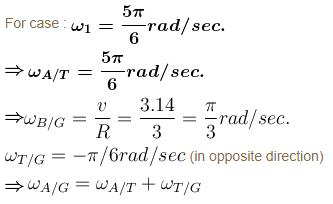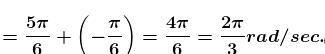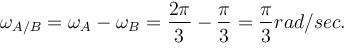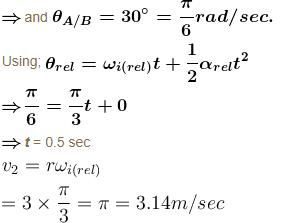The correct answers are: 0.5 sec, if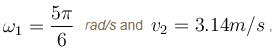A can not catch B within 0.5 sec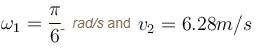*Multiple options can be correct
QUESTION: 8

A small sphere of mass m suspended by a thread is first taken aside so that the thread forms the right angle with the vertical and then released, then :

Solution: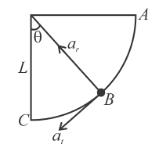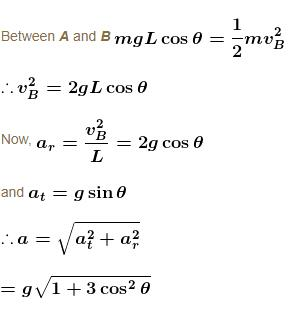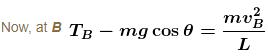By putting the value of VB

TB = 3mg cosθ

When total acceleration vector directed horizontally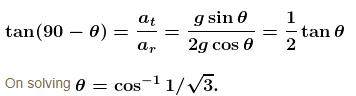The correct answers are: total acceleration of sphere as a function of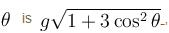thread tension as a function of θ is T = 3mg cos θ between the thread and the vertical at the moment when the total acceleration vector of the sphere is directed horizontal is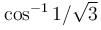*Multiple options can be correct
QUESTION: 9

A section of fixed smooth circular track of radius 20 m, in vertical plane is shown in the figure. A block is released from position A and leaves the track at B :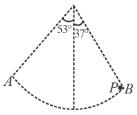Solution: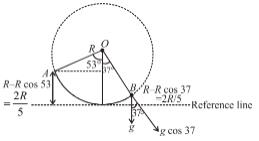By energy conservation between A and B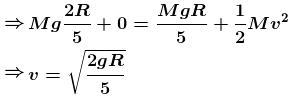Now, radius of curvature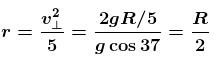The correct answers are: velocity at point B is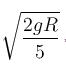Radius of curvature of trajectory when leaves point B is R/2

*Multiple options can be correct
QUESTION: 10

A particle is describing circular motion in a horizontal plane in contact with the smooth inside surface of a fixed right circular cone with its axis vertical and vertex down. The height of the plane of motion above the vertex is h and the semi-vertical angle of the cone is α. The period of revolution of the particle :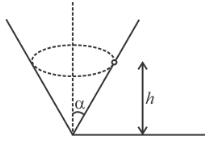Solution: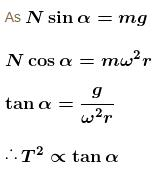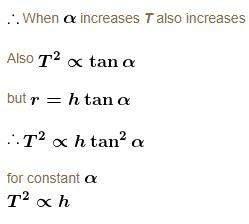Thus when h increases T also increases.
The correct answers are: increases as h increases, increases as α  increases